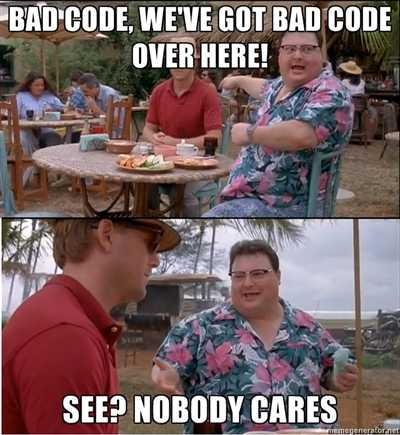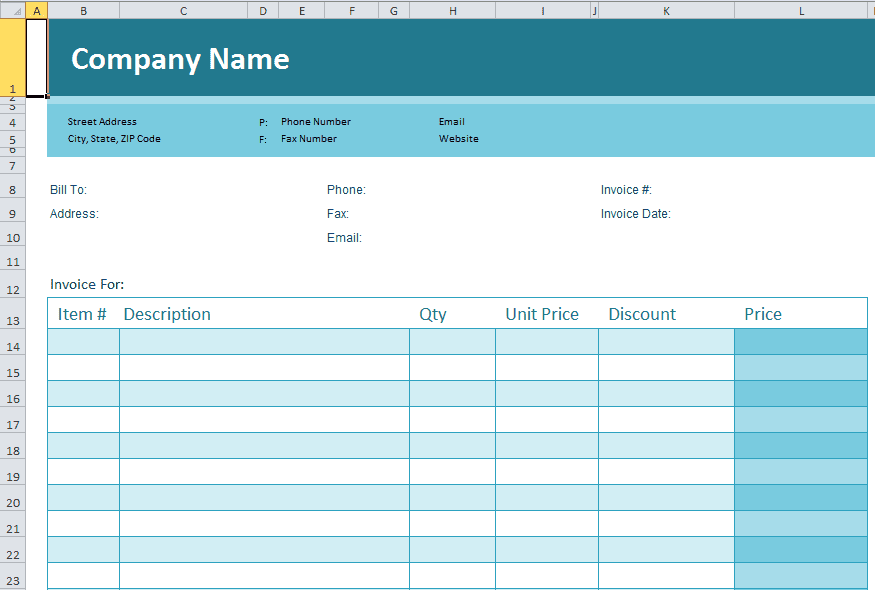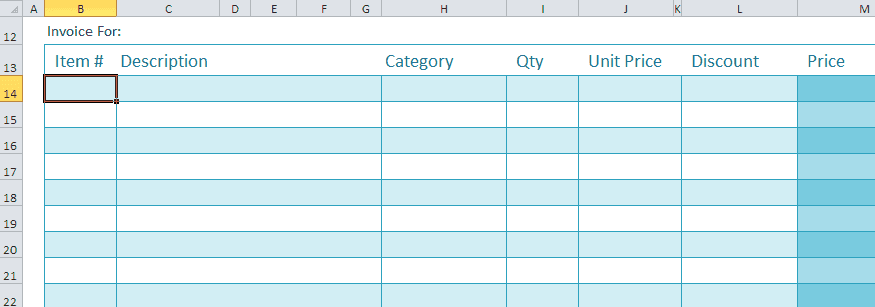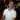# Using Enum to Keep Track of Worksheet Columns

May 13, 2013

If you code much in VBA, you have probably had to manipulate worksheets at some point. There are some common ways to work with worksheets through VBA. You have the Range object, where you can write in a string of the address you need to access. And you have the Cells object, where you use the [Row,Column] notation to access cells (and you can specify either numbers or strings for the columns). Some developers tend to use magic numbers, which they hard-codes the values into the code. While this can work, it’s really more of a hack job and it will most-likely make your life difficult down the line if you have to maintain the code. Using magic numbers has been deemed bad coding practice as quoted from Clean Code and I wholeheartedly agree.

In general it is a bad idea to have raw numbers in your code. You should hide them behind well-named constants.

And we all want to write good code, right? Regardless of how little some people care.Getting back to the topic: there is a clean, easily maintainable way to keep track of worksheet columns. And one way, is by using Enums.

## The Problem

Let’s say you were using this Excel template to create invoices.Notice that the Item# starts in Column B and Row 14. If you were to import data into this, you might use something like:

``````Option Explicit

Public Sub import()
Dim sh As Excel.Worksheet
Dim r As Excel.Range
Dim i As Long

Set sh = ThisWorkbook.Worksheets("Invoice")

Set r = AnotherWorkbook.Worksheets("Import Data").Range("A1:E10")

For i = 1 To r.Rows.Count
sh.Cells(13 + i, "B").Value = r.Cells(i, "A").Value
sh.Cells(13 + i, "C").Value = r.Cells(i, "B").Value
sh.Cells(13 + i, "H").Value = r.Cells(i, "C").Value
sh.Cells(13 + i, "I").Value = r.Cells(i, "D").Value
sh.Cells(13 + i, "K").Value = r.Cells(i, "E").Value
Next i
End Sub``````

This is a very simple procedure that imports data from one workbook into this Invoice workbook. Imagine that you had to reference the Invoice worksheet like this throughout hundreds of lines of code.

Then, say you added a column to the Invoice workbook called Category and you wanted it to go right after Description.Also, assume that the import range added the Category column to the column `F`.

Now you’ll have to go through all of your code and fix references made to the Description, Qty, Unit Price, and Discount columns. Your code would look like:

``````Public Sub import()
Dim sh As Excel.Worksheet
Dim r As Excel.Range
Dim i As Long

Set sh = ThisWorkbook.Worksheets("Invoice")

Set r = AnotherWorkbook.Worksheets("Import Data").Range("A1:F10")

For i = 1 To r.Rows.Count
sh.Cells(13 + i, "B").Value = r.Cells(i, "A").Value
sh.Cells(13 + i, "C").Value = r.Cells(i, "B").Value
sh.Cells(13 + i, "I").Value = r.Cells(i, "C").Value ' rename
sh.Cells(13 + i, "J").Value = r.Cells(i, "D").Value ' rename
sh.Cells(13 + i, "L").Value = r.Cells(i, "E").Value ' rename
sh.Cells(13 + i, "H").Value = r.Cells(i, "F").Value ' new col
Next i
End Sub``````

This can get particularly annoying if the sheet keeps changing over time. Plus, you may not always get every single reference updated, which can cause issues when you import/format the worksheet. And not to mention that it’s not very clear what Column I, J, L, and H are, so if you haven’t worked on your code in a while, you may have to click back-and-forth between the worksheet and your code to remember what they are.

So how do we fix this?## Using Enum to Track Worksheet Columns

One solution that I have been pretty happy about was to use Enum to keep track of Worksheet columns. If you’re not familiar with what Enums are, check out Chip Pearson’s website where he discusses the Enum Variable Type. He does a great job explaining the various uses of Enum.

Let’s start with our example from the beginning - before we added the column. We would create an enum like this:

``````Public Enum InvoiceCols
ItemNumber = 2  ' col B
Description = 3 ' col C
Qty = 8         ' col H
UnitPrice = 9   ' you get the idea
Discount = 11
End Enum``````

And we reference it in the code like:

``````Public Sub import()
Dim sh As Excel.Worksheet
Dim r As Excel.Range
Dim i As Long

Set sh = ThisWorkbook.Worksheets("Invoice")

Set r = AnotherWorkbook.Worksheets("Import Data").Range("A1:E10")

For i = 1 To r.Rows.Count
sh.Cells(13 + i, InvoiceCols.ItemNumber).Value = r.Cells(i, "A").Value
sh.Cells(13 + i, InvoiceCols.Description).Value = r.Cells(i, "B").Value
sh.Cells(13 + i, InvoiceCols.qty).Value = r.Cells(i, "C").Value
sh.Cells(13 + i, InvoiceCols.UnitPrice).Value = r.Cells(i, "D").Value
sh.Cells(13 + i, InvoiceCols.Discount).Value = r.Cells(i, "E").Value
Next i
End Sub``````

I really like how it actually makes the code much easier to understand as well. Rather than `"B"`, we can put `InvoiceCols.ItemNumber`, which makes it clear even to someone else that may manage the code later on.

Now let’s re-add the Category column. We have to update the Enum InvoiceCols like this:

``````Public Enum InvoiceCols
ItemNumber = 2  ' col B
Description = 3 ' col C
Category = 8    ' changed
qty = 9         ' changed
UnitPrice = 10  ' changed
Discount = 12   ' changed
End Enum``````

And the code would be updated like this:

``````Public Sub import()
Dim sh As Excel.Worksheet
Dim r As Excel.Range
Dim i As Long

Set sh = ThisWorkbook.Worksheets("Invoice")

Set r = AnotherWorkbook.Worksheets("Import Data").Range("A1:E10")

For i = 1 To r.Rows.Count
sh.Cells(13 + i, InvoiceCols.ItemNumber).Value = r.Cells(i, "A").Value
sh.Cells(13 + i, InvoiceCols.Description).Value = r.Cells(i, "B").Value
sh.Cells(13 + i, InvoiceCols.qty).Value = r.Cells(i, "C").Value
sh.Cells(13 + i, InvoiceCols.UnitPrice).Value = r.Cells(i, "D").Value
sh.Cells(13 + i, InvoiceCols.Discount).Value = r.Cells(i, "E").Value
sh.Cells(13 + i, InvoiceCols.Category).Value = r.Cells(i, "F").Value ' added
Next i
End Sub``````

All of the code that referenced the other columns can stay as they were, since they all reference the Enum’s value. You only have to add the code for the new column.

Wow, you read the whole article! You know, people who make it this far are true learners. And clearly, you value learning. Would you like to learn more about Excel? Please consider supporting me by buying me a coffee (it takes a lot of coffee to write these articles!).Written by Joseph who loves teaching about Excel.